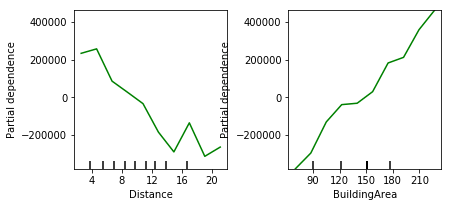#### partial dependence是什么意思？

统计/机器学习 监督式学习 特征选择    浏览次数：4972        分享

1

partial dependence是什么意思？有什么用？据说可以看特征和target的关系，然后选择特征，是这样的吗？桐桐酱   2018-09-23 12:39

##### 1个回答
8

Partial Dependence就是用来解释某个特征和目标值y的关系的，一般是通过画出Partial Dependence Plot(PDP)来体现。

PDP是依赖于模型本身的，所以我们需要先训练模型（比如训练一个random forest模型）。假设我们想研究$y$和特征$X_1$的关系，那么PDP就是一个关于$X_1$和模型预测值的函数。我们先拟合了一个随机森林模型$\text{RF}(X)$，然后用$X_{i}^k$表示训练集中第$k$个样本的第$i$个特征，那么PDP的函数就是

$$f(X_1)=\frac{1}{n}\sum_{k=1}^n\text{RF}(X_1, X_{2}^k,X_{3}^k,\cdots,X_{n}^k)$$SofaSofa数据科学社区DS面试题库 DS面经木子周   2018-10-31 09:18相关主题